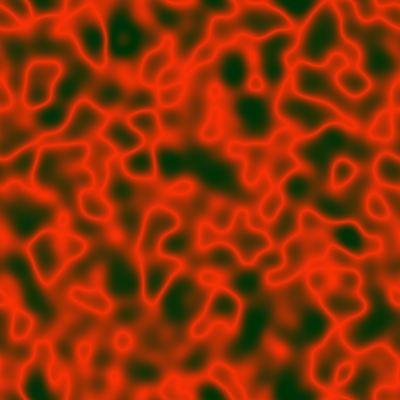Want to share your content on R-bloggers? click here if you have a blog, or here if you don't.

There’s a kind of error in the previous post. In this formula: $\exp\Bigl(-\frac{{(i/n-0.5)}^2 + {(j/n-0.5)}^2}{0.025^2} \Bigr),$ the indices $$i$$ and $$j$$ should range from $$0$$ to $$n$$, and $$n$$ must be even in order to get something centered. See the corrected code for details:

fplasma4 <- function(n = 400L, gaussianMean = -50, gaussianSD = 5) {
n <- n + 1L
M <- matrix(
rnorm(n*n, gaussianMean, gaussianSD),
nrow = n, ncol = n
)
FT <- dft(M)
n <- n - 1L
for(i in seq(n+1L)) {
for(j in seq(n+1L)) {
FT[i, j] <- FT[i, j] *
exp(-(((i-1L)/n - 0.5)^2 + ((j-1L)/n - 0.5)^2) / 0.025^2)
}
}
IFT <- dft(FT, inverse = TRUE)
colorMap1(IFT, reverse = c(FALSE, FALSE, TRUE))
}

The funny point is that the result is more interesting with the “error”. But it is not bad without it: